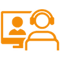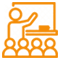# Maths Tricks

Learn speedy Maths tricks for fast calculation, addition, subtraction, multiplication, and division. These simple Maths tricks will help you to quickly solve maths problems and ace in Maths exams. Be it large or complex numbers, there are maths tricks for kids and adults to become a Mathematics Genius.## Best Maths Tricks For Fast Calculation

Here are Maths tricks and Maths Strategies which everyone should know and confidently solve Maths problems.

Adding large numbers like 744 + 338 can be a bit difficult. But if you know the Maths shortcut for addition, then adding can be easy through this easy Maths trick.

744 + 338

These numbers are definitely hard to contend with, rounding them up would make them easier to calculate. So, 744 becomes 750 and 338 becomes 340.

Now, add 750 and 340. The total is 1090. To find the answer to the actual equation, we need to find out how much we added to the numbers to round them up.

750 – 744 = 6 and 340 – 338 = 2

Now, add 6 and 2 together to make 8.

To find the answer to the original equation, we need to subtract 8 from 1090.

1090 – 8 = 1082

So the answer to 744 + 338 is 1082.

The rule which you need to follow while subtracting a large number from 1,000 is: Subtract every number except the last from 9 and subtract the final number from 10.

For example:

1,000 – 656

Step 1: Subtract 6 from 9 = 3

Step 2: Subtract 5 from 9 = 4

Step 3: Subtract 6 from 10 = 4

When we need to multiply the number 5 by an even number, use this maths shortcut to find the answer.

For example, 5 x 6 =

• Step 1: Cut the number being multiplied by five in to half. This makes the number 6 to be number 3.

5 x 6 = 30

When we need to multiply an odd number with 5, the formula is a bit different.

Let us consider 5 x 7.

• Step 1: Subtract one from the number that we need to multiply by 5, in this instance the number 7 becomes the number 6.
• Step 2: Now cut the number 6 into its half, which makes it the number 3. Make 5 the last digit. The number produced is 35, which is the answer.

5 x 3 = 15

The same can be applied to bigger odd and prime numbers.

The quick Maths trick to know when a number can be evenly divided by these certain numbers:

• 10 if the number ends in 0
• 9 when the digits are added together and the total is evenly divisible by 9
• 6 if the number is even and when you add the digits, the answer is evenly divisible by 3
• 8 if the last three digits are evenly divisible by 8 or are 000
• 5 if it ends in a 0 or 5
• 4 if the number ends with 00 or a two digit number that is evenly divisible by 4
• 3 when the digits are added together and the result is evenly divisible by the number 3
• 2 if it ends in 0, 2, 4, 6, or 8

This is an easy method that is helpful for multiplying any number by 9. Here is how it works:

Let’s use the example of 9 x 3.

Step 1: Subtract 1 from the number that is being multiplied by 9.

3 – 1 = 2

The number 2 is the first number in the answer to the equation.

Step 2: Subtract that number from the number 9.

9 – 2 = 7

The number 7 is the second number in the answer to the equation.

So, 9 x 3 = 27

Finding a percentage of a number is always a tricky process, but if you’ll think about it in the right terms, you’ll find that there’s a fast percentage finding trick which can save your time. For instance, to find out what 5% of 235 is, follow this method:

• Step 1: Move the decimal point over by one place, 235 becomes 23.5.
• Step 2: Divide 23.5 by the number 2, the answer is 11.75. That is also the answer to the original equation.

Let’s use the number 35 as an example.

• Step 1: Multiply the first digit by itself plus 1.
• Step 2: Put a 25 at the end.

35 squared = [3 x (3 + 1)] & 25

[3 x (3 + 1)] = 12

12 & 25 = 1225

35 squared = 1225

When multiplying large numbers, if one of the numbers is even, divide the first number in half, and then double the second number. This method will solve the problem quickly. For instance, consider

20 x 120

Step 1: Divide the 20 by 2, which equals 10. Double 120, which equals 240.

10 x 240 = 2400

The answer to 20 x 120 is 2,400.

Multiplying numbers that end in zero is actually quite simple. It involves multiplying the other numbers together and then adding the zeros at the end. For instance, consider:

200 x 400

Step 1: Multiply the 2 times the 4

2 x 4 = 8

Step 2: Put all four of the zeros after the 8

80,000

200 x 400= 80,000

Practicing these fast math tricks can help both students and teachers improve their math skills and become secure in their knowledge of mathematics—and unafraid to work with numbers in the future.

There are many more Maths Tricks which you should know. The tricks are:

#### Fake A Mystical Literary Connection With Anyone

At your local bookstore, you spot an attractive somebody carrying the exact same book as you; because it's the only book they sell (it's a bad bookstore). He or she seems a little skeptical when you say this means you must be soul mates, so you offer to prove it…...

#### Digital Roots: The Ultimate Mathematical Mind Game

There are many maths tricks that you can use to play math magic tricks. Learn the magic trick of digital roots. One time, lightning struck your computer, and your brain temporarily fused with the Internet. Your mind gained supercharged computation powers, but because it's the Internet, you only use them…...

#### Perfectly Sort Coins Without Looking At Them

Math has a lot in common with sorcery: Using vast sources of ancient knowledge passed from generations, it helps us compel the forces of nature to do our bidding just by scrawling out incomprehensible symbols on a whiteboard....

#### Amazing Tricks

Get the maths tricks for competitive exam and other short maths tricks.There are cases when you’re multiplying two numbers together and one of the numbers is even. In this case, you can divide that number by two and multiply the other number by 2....

#### Quickly Calculate The Weekday For Anyone's Birthday

How about you calculate the exact weekday of birth of your friend by just knowing their birth date, and that too in a matter of seconds. Well, if yes, then check this amazing Maths Maic trick to be a master....

#### Faster Percentage Calculation Tricks

If you really want to give your math skills a major boost, here are 11 most useful tricks that you will make you much better at math (or at least fake it 'till you make it!), all of which have kick-butt real-world applications....

#### 5 Maths Tricks

Mathematics tricks are a must for every one. They save crucial time and helps us in faster calculation and more. Browse below to get Maths Tricks with answers to become a better Mathematician....

#### Quick Mathematics Tricks

Mathematics Tricks always help us, whether in a school or during an exam. If you know how to add, subtract, divide and multiply fast, well, you are saving a lot of time for you. Check these mathematics ticks now....

#### Surprising Maths Tricks

A mathematics wizard has just discovered the largest known prime number and it's an astonishing 17 MILLION digits long. Curtis Cooper, of the University of Central Missouri, in the US found it by using hundreds of computers that were networked together....

#### 5 Time Saving Maths Tricks

There are cases when you’re multiplying two numbers together and one of the numbers is even. In this case you can divide that number by two and multiply the other number by 2. You can keep doing this until you get numbers that are easy to work with in your…...

## Explore

#### SchoolPlus Program

Yearlong program for Olympiads preparation & to build necessary skills for future.

Explore MoreExplore MoreExplore MoreAssess your performance by taking topic-wise and full length mock tests.

Explore MoreExplore MoreExplore More